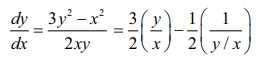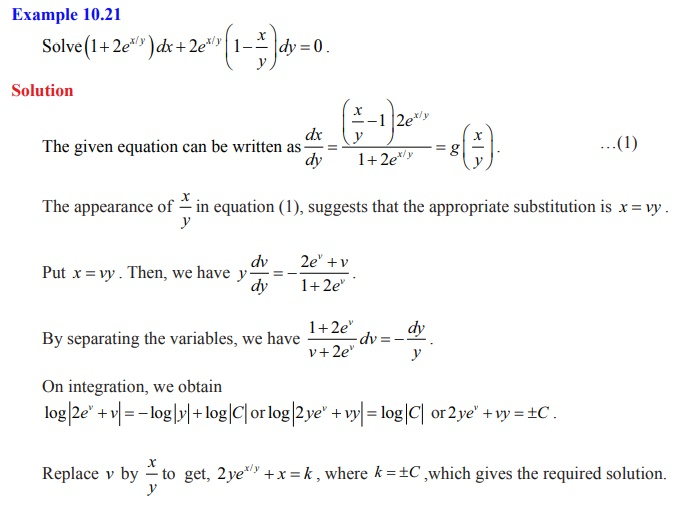Home | | Maths 12th Std | Homogeneous Form or Homogeneous Differential Equation

# Homogeneous Form or Homogeneous Differential Equation

Solution of First Order and First Degree Differential Equations: Homogeneous Form or Homogeneous Differential Equation

## Solution of First Order and First Degree Differential Equations

Homogeneous Form or Homogeneous Differential Equation

### Definition 10.12 : (Homogeneous Function of degree n)

A function f ( x, y) is said to be a homogeneous function of degree n in the variables x and y if, f (tx , ty ) = t n f ( x, y) for some n for all suitably restricted x , y and t . This is known as Euler’s homogeneity.

For instance,

(i) f (x, y) = 6x2 + 2xy + 4 y2 is a homogeneous function in x and y, of degree two.

(ii) But f (x, y) = x3 + ( sin x)ey is not a homogeneous function.

If f ( x, y) is a homogeneous function of degree zero, then there exists a function g such that f ( x, y) is always expressed in the form g(y/x) or g(x/y).

### Definition 10.13: (Homogeneous Differential Equation)

An ordinary differential equation is said to be in homogeneous form, if the differential equation is written as dy/dx = g(y/x) .### Caution

The word “homogeneous” used in Definition 10.7 is different from in Definition 10.12.

### Remark

(i) The differential equation M ( x, y)dx + N( x, y)dy = 0 [in differential form] is said to be homogeneous if M and N are homogeneous functions of the same degree.

(ii) The above equation is also written as dy/dx = f ( x, y) [in derivative form] where

f ( x, y) = −M ( x, y) / N ( x, y) is clearly homogeneous of degree 0 .

For instance

(1) consider the differential equation (x2 3y2)dx + 2xy dy = 0 . The given equation is rewritten as. Thus, the given equation is expressed asHence, (x2 - 3y2)dx + 2xydy = 0 is a homogeneous is a homogeneous is a homogeneous differential equation.

(2) However, the differential equationis not homogeneous. (verify!)

To find the solution of a homogeneous differential equation dy/dx = g(y/x), consider the substitution v = y/x. Then, y = xv and dy/dx = v + x dv/dx .Thus, the given differential equation becomes x dv/dx = f (v) v which is solved using variables separable method. This leads to the following result.

### Theorem 10.1

If M ( x, y ) dx + N( x , y)dy = 0 is a homogeneous equation, then the change of variable y = vx, transforms into a separable equation in the variables v and x .Tags : Solution of First Order and First Degree Differential Equations | Mathematics , 12th Maths : UNIT 10 : Ordinary Differential Equations
Study Material, Lecturing Notes, Assignment, Reference, Wiki description explanation, brief detail
12th Maths : UNIT 10 : Ordinary Differential Equations : Homogeneous Form or Homogeneous Differential Equation | Solution of First Order and First Degree Differential Equations | Mathematics